If you need to provide overloaded operators in traditional, unmanaged C++, you’ll find that operator overloading is done rather differently. Operator overloads use the operator keyword to name an overload function, so the addition operator is implemented by a function named operator+, the equality operator by one named operator==, the increment operator by one named operator++, and so on. These functions can either be member functions of the type or global functions that aren’t a member of any type.

There’s a lot more to operator overloading in unmanaged C++. If you need to know more, consult a book or tutorial on C++ programming.

8.        Try compiling your program again. The following output should be expected.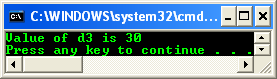You should find that the compilation is successful this time because the compiler recognizes that you have implemented the + operator. If you run the program, you should get the answer 30.0 printed out because of the following two lines:

Console::Write("Value of d3 is ");

Console::WriteLine(d3.getVal());

If your type implements an operator, the compiler maps C++ operators (such as +) onto the equivalent function for you. You can also call the operator function explicitly, although there’s generally little reason why you’d want to do so. So, you could replace the addition line, as follows:

// d3 = d1 + d2;

d3 = Dbl::operator+(d1, d2);

A static method is accessed by using the type name followed by the scope (::) operator and the name of the function. Now that you’ve seen how to implement the addition operator, it will be simple for you to implement the other arithmetic operators for the struct (subtraction, division, multiplication, and modulus).

d3 = d1 + d2;

// Add a Dbl and an int

d3 = d1 + 5;

// Add an int and a Dbl

d3 = 5 + d2;

You can add overrides of the operator+ function to handle this task. The following short exercise shows you how.

1. Continue using the project from the previous exercise.

2. Find the operator+ function in the Dbl struct. Add the following two variations after it:

// Adding a Dbl and an int

static Dbl operator+(Dbl lhs, int rhs)

{

Dbl result(lhs.val + rhs);

return result;

}

// Adding an int and a Dbl

static Dbl operator+(int lhs, Dbl rhs)

{

Dbl result(lhs + rhs.val);

return result;

}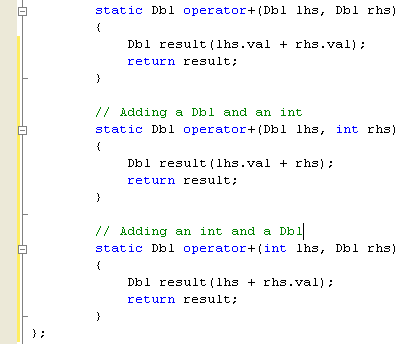3.   Add two more additions to your main() function to test the new operator functions, plus some calls to Console::WriteLine to verify that the additions have worked correctly.

d3 = d1 + d2;

Console::Write("d3 = d1 + d2, value of d3 is ");

Console::WriteLine(d3.getVal());

// Add a Dbl and an int

d3 = d1 + 5;

Console::Write("d3 = d1 + 5, value of d3 is now ");

Console::WriteLine(d3.getVal());

// Add an int and a Dbl

d3 = 5 + d2;

Console::Write("d3 = 5 + d2, value of d3 is now ");

Console::WriteLine(d3.getVal());

4.        Now for the interesting step: remove (or comment out) those last two operator overloads you added in the previous step, and then recompile the code and run it.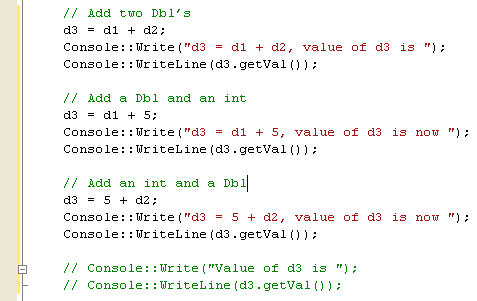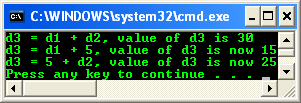You should find that it works exactly the same as it did with the operators left in. So what’s going on? You can find out by using the debugger.

5.   Place a breakpoint in the code by clicking in the gray margin to the left of the code, next to the d3 = d1 + 5 line. You should see a red dot in the margin, showing that a breakpoint has been set.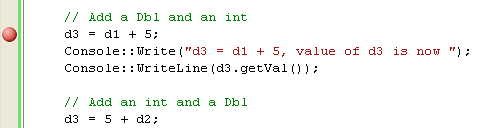6.   Run the program by pressing the F5 key. The program will start up and then stop at the breakpoint. Now press the F11 key to step into the next function to be executed. You might expect this function to be operator+, but you’d be wrong because you step into the Dbl constructor.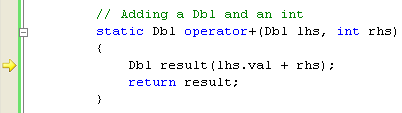7.  Press F11 again, and you’re finished with the constructor and back to the d3 = d1 + 5 line. Press it once more, and you’re in operator+, where you might expect to be.

Why did you get an extra call to the Dbl constructor? Because the compiler saw an integer in the code, but it only has an operator+ function that takes two Dbl values. However, because the Dbl struct has a constructor that takes a double, the compiler has cast the integer as a double and then used the constructor to create a temporary Dbl object to pass to the function. When you’re in the operator+ function, if you examine the arguments in the Locals window, you’ll see that they are both of type Dbl and that the rhs argument has the value 5.0.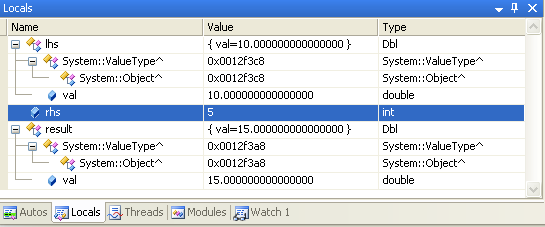You can see that, provided your types implement the correct constructors, you can sometimes use one operator overload to perform several different conversions.